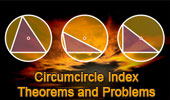# Plane Geometry: Circumcircle, Circumradius of a triangle, Theorems and Problems 6

 Circumcircle of a triangle - Table of Content 6Steiner Theorem. Circumcircle, Circumcenter, circumradius, inradius, exradii Geometry Online Glossary . Geometry Glossary based on the new New York State mathematics standards initiative. Right Triangle: Circumcenter. Centers, Inradius, Circumradius, Incenter, Circumcenter, Orthocenter, Centroid, Poncelet's theorem, Sagitta. Proposed Problem 160. Triangle, Incircle, Incenter, Circumcircle, Circumcenter, Inradius. Proposed Problem 159. Circumcircle, Distances from the Circumcenter to the Incenter and the Excenters. Proposed Problem 158. Circumcircle, Circumcenter  Relation between the Circumradius, Inradius and Exradii of a triangle. Proposed Problem 157. Circumcircle, Distance from the Circumcenter to the Excenter. Proposed Problem 156. Circumcircle, Circumcenter Triangle, Circumradius, Exradius, Chord, Secant line. Proposed Problem 155. Euler's Theorem: Distance from the Incenter to the Circumcenter. Circumcircle. Proposed Problem 154. Circumcircle, Circumcenter. Triangle, Inradius, Circumradius, Chord. Go to page: Previous | 1 | 2 | 3 | 4 | 5 | 6 | 7 | 8 | Next

 Home | Search| Geometry | Triangles | Centers | Post a comment | Email | By Antonio Gutierrez